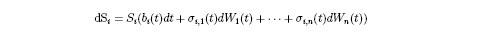# Stochastic Portfolio Optimization, from Chris Hammond

November 19, 2008 |The Math Department at the University of Michigan has held an annual lecture series called the Ziwet Lectures since 1936. Past speakers include von Neuman, Kac, Thurston, and about a half a dozen Fields medalists. This year, the speaker is I. Karatzas . He is giving a series of three lectures. Today he discussed Stochastic Portfolio Optimization (the next lectures will be on Volatility and Arbitrage respectively). He spent a lot of time introducing the subject, which was good for me. One assumes that there are n risky assets available, S_1, . . .,S_n, and they evolve according to the stochastic differential equationwhere dW_i(t) is Brownian motion. X(t) is one's wealth at time t and p_i(t) is the percent of one's wealth invested in asset i at time t. Denote by p=(p_1,…,p_n) our portfolio. U(x) is a utility function, i.e., any increasing function that is concave down. Our goal is to maximize the expected value of utility at the time T. In other words, we let V(x)=sup{E[U(X(T)]} where the supremum is taken over all possible portfolios given that our initial wealth was X(0)=x. Apparently we are guaranteed existence of such a thing in general, but finding the optimal strategy is not very tractable, so as always, one starts with special cases.

If we assume that the utility function is U(x)=log(x) or U(x)=x^{a}/a for 0<a<1, then one can find a reasonable solution. However, the solution depends on having reliable values for sigma_{i,j}(t) for all times t as well as for the interest rate.

If one assumes that all coefficients involved are constant, then we can handle the problem of a general utility function. The solution is characterized by a partial differential equation called the Hamilton-Jacobi-Bellman (HJB ) equation. Because we have assumed U is concave down, we can apply the Legendre transform and linearize the partial differential equation. We can then solve the linearized equation.

Karatzas ended the talk with several open problems.

I am not sure whether this lends itself directly to practical application, but perhaps it inspires some more practical ideas.

Why would one assume the coefficients are constant?

## Chris Hammond responds:

One answer is that over a reasonably short time horizon, they would be approximately constant. I think the same question could be asked of the Black-Scholes model. It is assumed that if S is the price of an asset, dS=S*r*dt+S*sigma*dW(t), where r is the expected return on the asset, sigma is its volatility, and W(t) is Brownian motion. More sophisticated models assume that the volatility is also a random variable that changes with time sigma=sigma(t). But it makes sense to start with the simpler, constant, case.

In some situations in math, it is insightful to assume very simple behavior to get a model case and view reality as some sort of perturbation of that.

I am not sure it is a good answer, but I'm trying to learn more about these things, so if I find a more satisfying answer, I'll let you know.